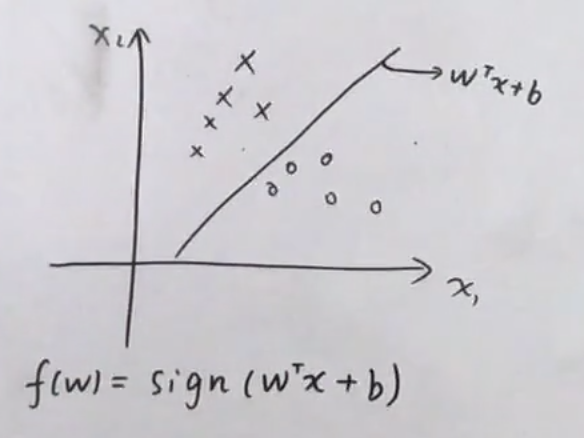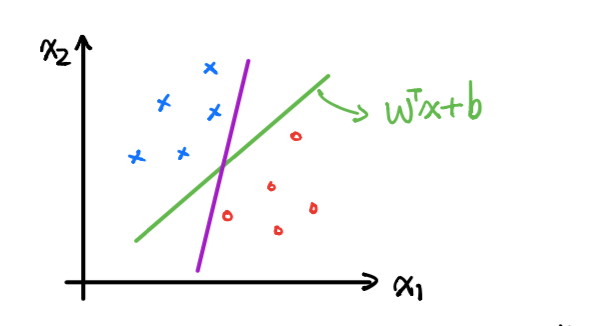SVM is a very very important machine learning althogrim. Since it is basic and logical, it frequently appears in Deep Learning Engineering Interview. However, it is not a kind of Deep Learning method. It does not need a large number of training data. Before the deep learning method becomes popular, it is the only superstar in the machine learning area.

# Key Points

There are three key points for SVM. They are souls of this method. They are, 1. Margin 2. Duality 3. Kernel Function a. KKT  b. slater We will discuss them later in deeply. There are three kinds of SVM. They are: Hard-margin SVM Soft-margin SVM Kernel SVM.

# Hard-margin SVM

SVM was proposed for addressing Binary classification problem, as shown in Fig 1.We denote a hyperplane $$w^t x + b$$ and the classfication function is $$f(x) = sign(w^tx,b)$$, which is based on the discriminative model, not probility model. In the space, there are initfe unlimited number of the hyperplane. The purple line is an example.Obviously, it is not a good choice, since it is sensitive to noise. The key idea of the SVM is to find the best the hyperplane to clssifiy the points, which have the max margin for each kinds of point.

## Show in Math

We donte the point as $${x^i,y^i}$$ where $x^i R$ and $$y^i \in {-1,1}$$. The max margin ($$w, b$$), which we want to find, is